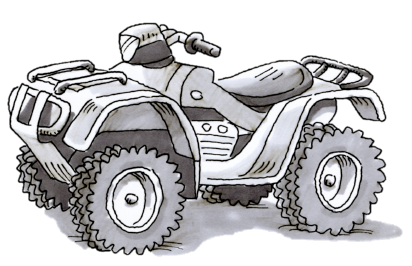### Home > ACC7 > Chapter 8 Unit 9 > Lesson CC3: 8.1.2 > Problem8-20

8-20.

Jack noticed a sale on his favorite model of All-Terrain Vehicle (ATV). The salesperson said the company was cutting the price for that weekend, so Jack got an $8\%$ discount.

1. If the usual price of the ATV was $4298$, estimate how much the discount will be. Explain your reasoning.

Round the ATV price to $4300$ in order to make the percent easier to approximate.

Since we can find that $10\%$ of $4300$ is $430$, we can also determine that $5\%$ of $4300$ would be $215$. $8\%$is roughly in the middle of $10\%$ and $5\%$. What is your estimation?

2. Calculate the amount Jack did pay with the $8\%$ discount.

Find $8\%$ of $4298$, then subtract that value from $4298$ to find how much Jack paid.

$4298 − 343.84 =$

$4298\left(.08\right) = 343.84$

$3954.16$1. If the store sold three of the ATVs that weekend, how much money was saved by the three customers combined?

For each ATV, the original price was $4298$. Based on part (b), it costs $3954$.16 after the $8\%$ discount. How much did Jack save on one ATV? Now multiply that number by $3$.